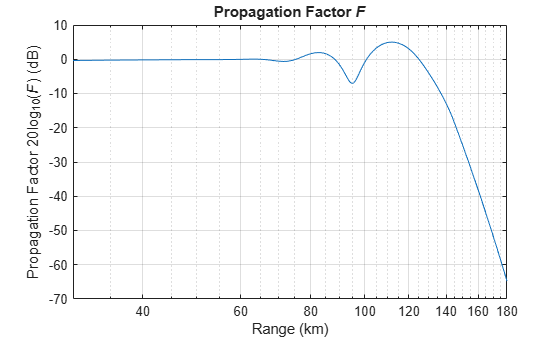## Syntax

``F = radarpropfactor(R,freq,ANHT)``
``F = radarpropfactor(___,TGTHT)``
``F = radarpropfactor(___,Name,Value)``
``radarpropfactor(___)``

## Description

````F = radarpropfactor(R,freq,ANHT)` calculates the one-way propagation factor assuming a surface target and a sea state of `0`. The calculation estimates the complex relative permittivity (dielectric constant) of the reflecting surface using a sea water model described in  that is valid from `100` MHz to `10` GHz. The target height is assumed to be the height of significant clutter sources above the average surface height. Specifically, the target height is calculated as `3` times the standard deviation of the surface height. Assuming the paths are the same, the two-way propagation factor is 2`F`.```
````F = radarpropfactor(___,TGTHT)` calculates the target propagation factor assuming a target height of `TGTHT`.```

example

````F = radarpropfactor(___,Name,Value)` allows you to specify additional input parameters as Name-Value arguments. You can specify additional name-value pair arguments in any order as `Name1`,`Value1`,...,`NameN`,`ValueN`. This syntax can use any of the input arguments in the previous syntax.```
````radarpropfactor(___)` plots the one-way propagation factor in dB versus range in km. Default range units are km.```

## Examples

collapse all

Plot the propagation factor for a `3` GHz S-band radar assuming an antenna height of 10 m and a target height of `1` km. Assume that the surface has a height standard deviation of `1` m, and the surface slope is `0.05` degrees.

```R = (30:0.5:180)*1e3; % Range (m) freq = 3e9; % Frequency (Hz) anht = 10; % Radar height (m) tgtht = 1e3; % Target height (m) hgtsd = 1; % Height standard deviation (m) beta0 = 0.05; % Surface slope (deg) radarpropfactor(R,freq,anht,tgtht,... 'SurfaceHeightStandardDeviation',hgtsd,... 'SurfaceSlope',beta0)```## Input Arguments

collapse all

Free space range, specified as a scalar or an M-length vector. Units are in meters.

Example: `0.5`

Data Types: `single` | `double`

Radar frequency in hertz, specified as a positive real scalar or a vector.

Data Types: `double`

Antenna height as referenced from ground level, specified as a positive scalar. Units are in meters.

Data Types: `double`

Target height as referenced from ground level, specified as a positive scalar. Units are in meters.

Data Types: `double`

### Name-Value Pair Arguments

Specify optional comma-separated pairs of `Name,Value` arguments. `Name` is the argument name and `Value` is the corresponding value. `Name` must appear inside quotes. You can specify several name and value pair arguments in any order as `Name1,Value1,...,NameN,ValueN`.

Example: `'SurfaceHeightStandardDeviation',hgtsd,'SurfaceSlope',beta0`

Polarization of the transmitted wave, specified as `'H'` or `'V'`. `'H'` indicates horizontal polarization and `'V'` indicates vertical polarization.

Complex relative permittivity (dielectric constant) of the reflecting surface, specified as a complex scalar. The default value of dielectric constant depends on the value of the `freq` argument. The function uses a sea water model in  that is valid up to `10` GHz.

Data Types: `single` | `double`
Complex Number Support: Yes

Standard deviation of the surface height in meters, specified as positive scalar. The default value of `0.01` m indicates a sea state of `0`. Units are in meters.

Data Types: `single` | `double`

Surface slope, specified as a nonnegative scalar. This value is expected to be 1.4 times the RMS surface slope. Given the condition that 2*GRAZ/BETA0 < 1, where GRAZ is the grazing angle of the geometry specified in degrees, the effective surface height standard deviation in meters is calculated as

Effective HGTSD = HGTSD*(2*GRAZ/BETA0)^(0.2)

This calculation better accounts for shadowing. Otherwise, the effective height standard deviation is equal to HGTSD. BETA0 defaults to the surface slope value output by the `searoughness` function for a sea state of `0`. Units are in degrees.

Data Types: `single` | `double`

Surface vegetation type, specified as `'Trees'`, `'Weeds'`, and `'Brush'` are assumed to be dense vegetation. `'Grass'` is assumed to be thin grass. Use this argument when using the function on surfaces different from the sea.

Half-power elevation beamwidth, specified as a scalar between 0° and 90°. The elevation beamwidth is used in the calculation of a `sinc` antenna pattern. The default antenna pattern is symmetrical with respect to the beam maximum and is of the form sin(u)/u. The parameter u is given by u = k*sin(theta), where theta is the elevation angle in radians and k is given by k = 1.39157/sin(ELBW/2). Units are in degrees.

Data Types: `double`

Antenna elevation pattern, specified as an M-length vector. This is an alternative to specifying the elevation beamwidth. Both `'AntennaPattern'` and `'PatternAngle'` arguments must be vectors of the same size. If both an antenna pattern and elevation beamwidth are provided, the function uses the antenna pattern and ignores the elevation beamwidth value. Defaults to a `sinc` antenna pattern.

Data Types: `double`

Antenna pattern angle, specified as an M-length vector corresponding to the `'AntennaPattern'` argument. In general, to properly compute the coverage, the pattern should be specified from `-90°` to `90°`. Units are in degrees.

Data Types: `double`

Tilt angle of the antenna with respect to the surface, specified as a scalar between `-90°` and `90°`. Units are in degrees.

Data Types: `double`

Effective Earth radius, specified as a positive scalar. The default value calculates the effective Earth radius using a refraction gradient of `-39e-9`, which results in approximately `4/3` of the real earth radius. Units are in meters.

Data Types: `double`

Refractive index at the surface, specified as a nonnegative scalar. Defaults to approximately `1.000318`, which is the output of the `refractiveidx` function at an altitude of `0` meters.

Data Types: `double`

## Output Arguments

collapse all

The one-way propagation factor, returned as a scalar or M-length column vector. Units are in decibels.

 Blake, L.V. "Machine Plotting of Radar Vertical-Plane Coverage Diagrams." Naval Research Laboratory, 1970 (NRL Report 7098).